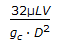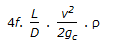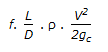# Chemical Engineering - Fluid Mechanics

1.
One poise (unit of absolute/dynamic viscosity) is equivalent to one
gm/cm2. sec.
gm/cm. sec.
cm2/sec.
m2/sec.
Explanation:
No answer description is available. Let's discuss.

2.
The equation relating friction factor to Reynold number, f-0.5 = 4 loge (NRe/f)-0.4, is called the __________ equation.
Von-Karman
Blausius
Colebrook
Explanation:
No answer description is available. Let's discuss.

3.
Pressure drop for turbulent fluid flow through a circular pipe is given by
64/ReExplanation:
No answer description is available. Let's discuss.

4.
What is the co-efficient of contraction, if a fluid jet discharging from a 50 mm diameter orifice has a 40 mm diameter at its vena-contracta ?
0.64
1.65
0.32
0.94